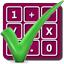#### Arithmetic of Computers

#### from Tenscope Limited

By using this site, you are accepting "session" cookies, as set out in the site's Privacy Policy
Cookies are also used to remember which page of the book you last viewed, so that when you revisit the site you automatically return to the last page you visited.

### Lesson 1

#### Page 37

Your answer :
I don’t get this “b” and “n” business.
Sometimes it is confusing to substitute numbers for letters, and vice versa. But the ability to do so is very important in learning mathematics, and luckily it comes with experience.
Now, you understand what it means to raise a number to a certain power. The number 6 raised to the second power simply means 62 or 6 × 6, and 62 = 36.
In this equation, 6 is called the base and 2 is called the exponent. The general formula for writing the power of any number, therefore, is
BASE(exponent)
or, since we are going to let b stand for base and n stand for exponent,
bn
Now, the expression, bn, can be used to make general statements about powers of numbers. But when you are asked to use specific numbers for b and n, you simply substitute them for the letters of the general symbol. Thus if b = 5, and n = 3, then
bn = 53
Now return to Page 17 and pick the correct answer.

Answer to Self-Test Question 11, Lesson 1 :
42 or 4 raised to the second power.